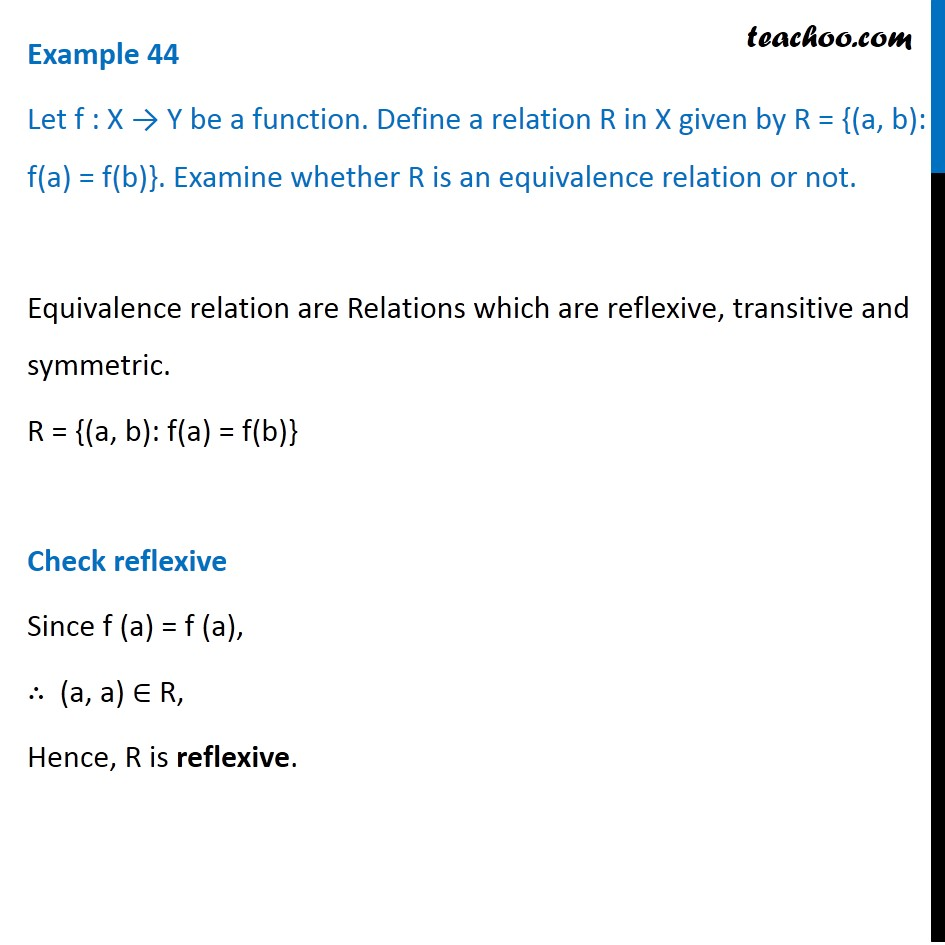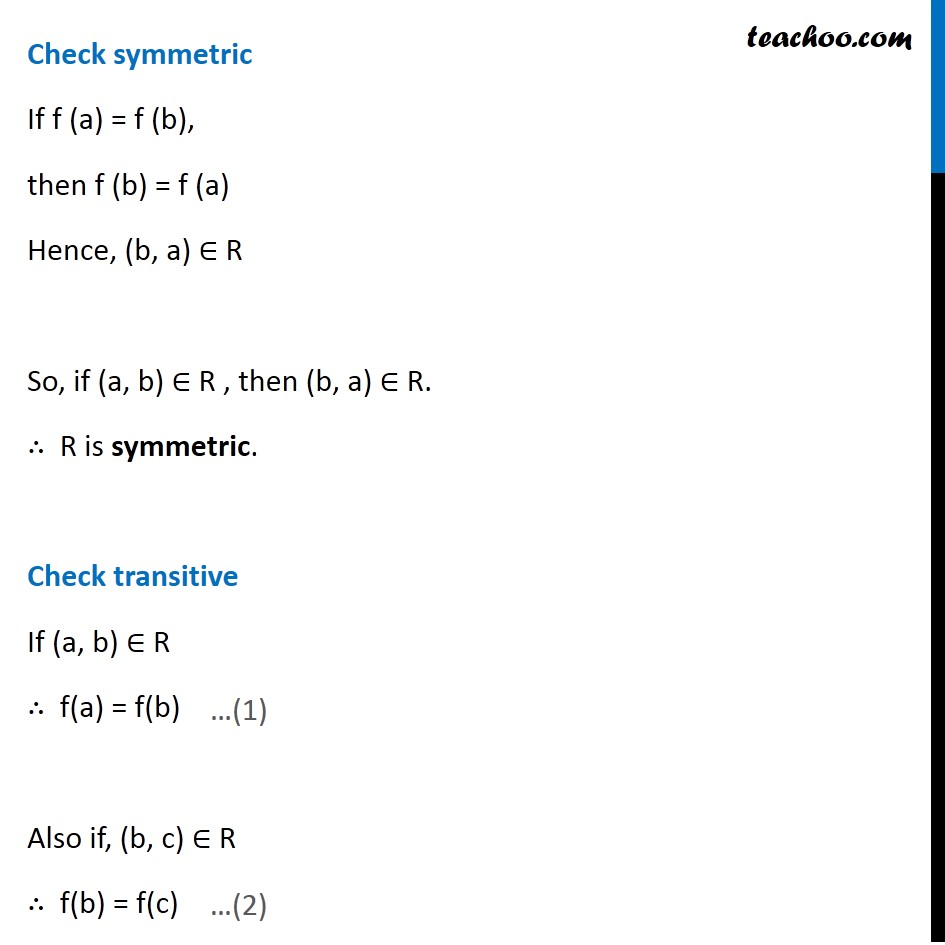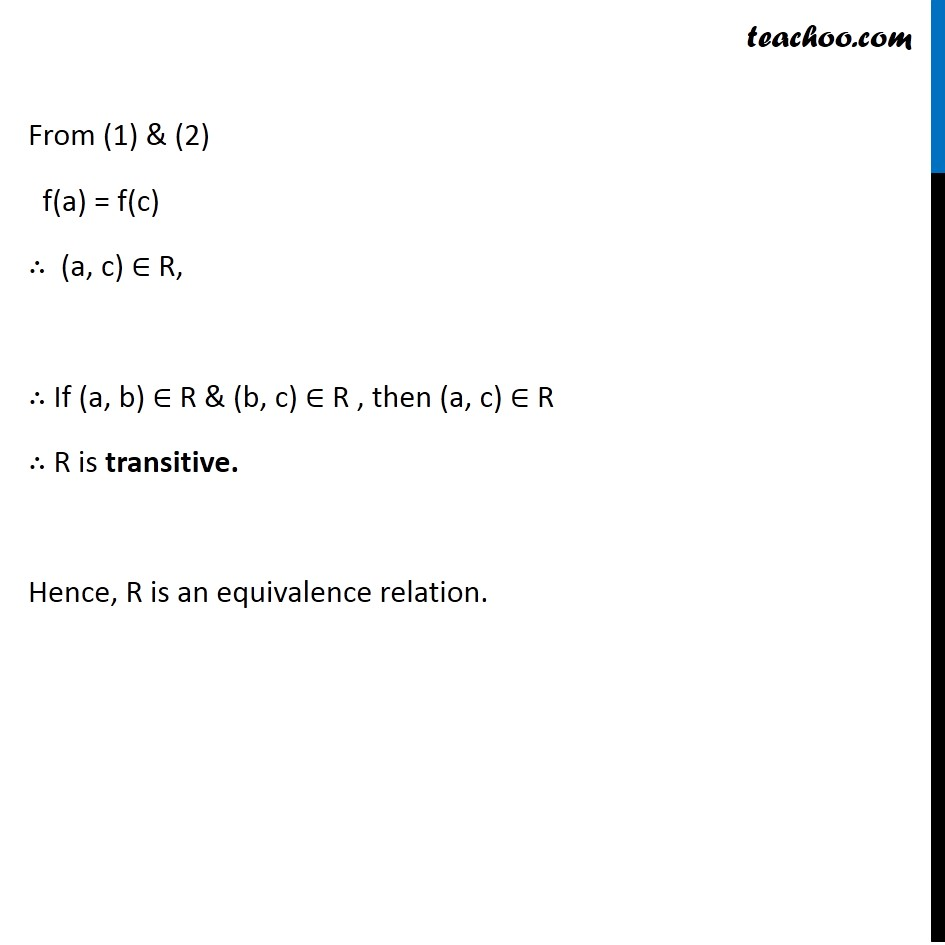Examples

Chapter 1 Class 12 Relation and Functions
Serial order wiseLearn in your speed, with individual attention - Teachoo Maths 1-on-1 Class

### Transcript

Example 21 Let f : X → Y be a function. Define a relation R in X given by R = {(a, b): f(a) = f(b)}. Examine whether R is an equivalence relation or not. Equivalence relation are Relations which are reflexive, transitive and symmetric. R = {(a, b): f(a) = f(b)} Check reflexive Since f (a) = f (a), ∴ (a, a) ∈ R, Hence, R is reflexive. Check symmetric If f (a) = f (b), then f (b) = f (a) Hence, (b, a) ∈ R So, if (a, b) ∈ R , then (b, a) ∈ R. ∴ R is symmetric. Check transitive If (a, b) ∈ R ∴ f(a) = f(b) Also if, (b, c) ∈ R ∴ f(b) = f(c) From (1) & (2) f(a) = f(c) ∴ (a, c) ∈ R, ∴ If (a, b) ∈ R & (b, c) ∈ R , then (a, c) ∈ R ∴ R is transitive. Hence, R is an equivalence relation.